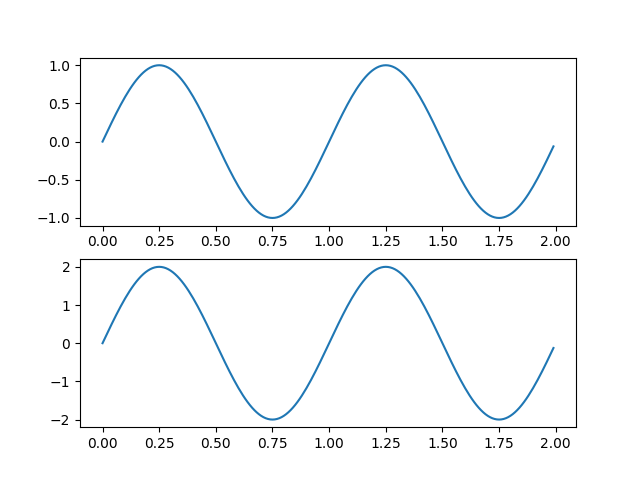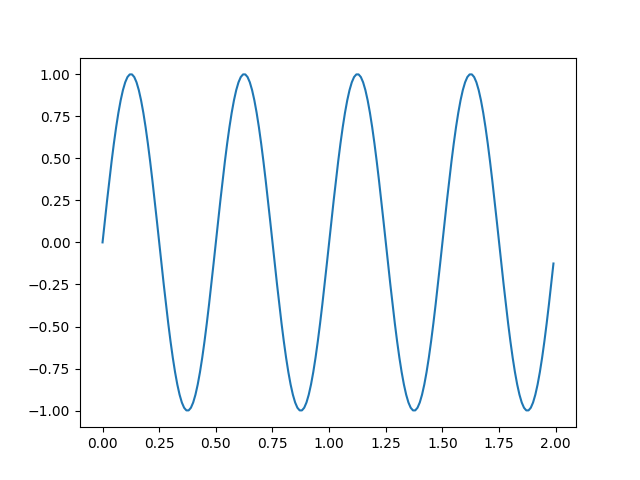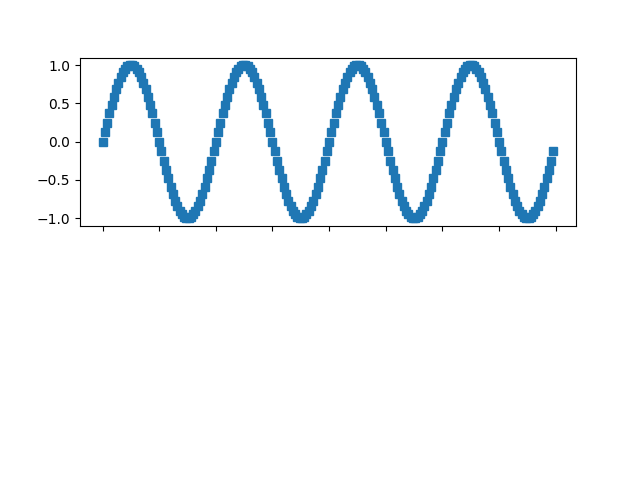# Managing multiple figures in pyplot#

matplotlib.pyplot uses the concept of a current figure and current axes. Figures are identified via a figure number that is passed to figure. The figure with the given number is set as current figure. Additionally, if no figure with the number exists, a new one is created.

Note

We discourage working with multiple figures in pyplot because managing the current figure is cumbersome and error-prone. Instead, we recommend to use the object-oriented approach and call methods on Figure and Axes instances.

import matplotlib.pyplot as plt
import numpy as np

t = np.arange(0.0, 2.0, 0.01)
s1 = np.sin(2*np.pi*t)
s2 = np.sin(4*np.pi*t)


Create figure 1

plt.figure(1)
plt.subplot(211)
plt.plot(t, s1)
plt.subplot(212)
plt.plot(t, 2*s1)Out:

[<matplotlib.lines.Line2D object at 0x7fb682379f00>]


Create figure 2

plt.figure(2)
plt.plot(t, s2)Out:

[<matplotlib.lines.Line2D object at 0x7fb68265e860>]


Now switch back to figure 1 and make some changes

plt.figure(1)
plt.subplot(211)
plt.plot(t, s2, 's')
ax = plt.gca()
ax.set_xticklabels([])

plt.show()Total running time of the script: ( 0 minutes 1.082 seconds)

Keywords: matplotlib code example, codex, python plot, pyplot Gallery generated by Sphinx-Gallery